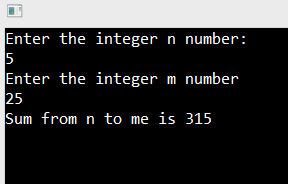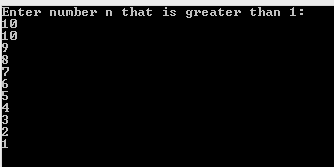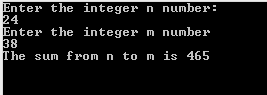In this article, we are going to learn how to use loops in C#, what type of loops exist, and when to use them. We will use a couple of examples to closely explain the usage of each loop in C#.

For the complete navigation of this series check out: C# Back to Basics.

If you want to download the source code for our examples, you can do that from here Loops in C# Source Code.

Let’s dig in.

## While Loop

While loop is a loop with a precondition. This means that we are checking a condition first and then if a condition returns true, we execute our expression:

```while(condition)
{
< expression > ;
}
```

Example 1: Create an application which calculates the sum of all the numbers from n to m (inputs from a user):
```class Program
{
static void Main(string[] args)
{
Console.WriteLine("Enter the integer n number:");

Console.WriteLine("Enter the integer m number");

int sum = 0;

while(n <= m)
{
sum += n;
n++;
}

Console.WriteLine(\$"Sum from n to me is {sum}");
}
}
```So let’s explain the code above.

Because we calculate the sum of all numbers from “n” to “m”, we need to have a variable to store that value. It needs to be initialized with a zero at a beginning. Without that, our app will fail to build due to the sum variable being unassigned.

In a while loop, we are going through all the numbers from n to m and adding every number to the sum variable. We are using this expression: sum += n; which is a shorter for sum = sum + n;

Finally, we need to increment the n variable by 1. Without that, we would have an infinite loop because the value of the n variable would always be lesser than the value of the „m“ variable.

We should use while loops when the number of iterations is uncertain. This means that we could repeat iteration until some condition is fulfilled, but we are not sure how many iterations we would need to reach the condition fulfillment.

## For Loop

For loop is another loop with a precondition. We use the following syntax to write it in C#:

```for (initialization; condition; progression;)
{
<loop body > ;
}
```

We use initialization at the beginning of the loop and it serves the purpose of initializing the variable with a value. The condition is used to determine when the loop is completed. Progression is a part in which we increment or decrement our variable initialized in the initialization part. The body consists of all the expressions we need to execute as long as the condition is true.

It is important to know that the order of execution is: Initialization, Condition, Loop Body, Progression.

Example 1: Create an application which calculates the sum of all the numbers from n to m (inputs from a user):

```class Program
{
static void Main(string[] args)
{
Console.WriteLine("Enter the integer n number:");

Console.WriteLine("Enter the integer m number");

int sum = 0;

for(int i = n; i <= m; i++)
{
sum += i;
}

Console.WriteLine(\$"Sum from n to m is {sum}");

}
}
```Example 2:  Create an application that prints out all the integer numbers from n to 1:

```class Program
{
static void Main(string[] args)
{
Console.WriteLine("Enter number n that is greater than 1: ");

for (int i = n; i >= 1; i--)
{
Console.WriteLine(i);
}

}
}
```We should use for loops when we know how many iterations we are going to have. This means if we iterate through all the elements inside a collection or we have an ending point for iterations.

## Do-While Loop

The do-while loop is a loop with postcondition. What this means is that the loop body is executed first and the condition is checked after. That’s totally opposite from the previous loop examples.

We can implement this loop in the following way:

```do
{
< expression > ;
} while (condition);
```

Example 1: Create an application which calculates the sum of all the numbers from n to m (inputs from a user):
```class Program
{
static void Main(string[] args)
{
Console.WriteLine("Enter the integer n number:");

Console.WriteLine("Enter the integer m number");

int sum = 0;

do
{
sum += n;
n++;
} while (n <= m);

Console.WriteLine(\$"The sum from n to m is {sum}");
}
}
```Example 2: Create an application which prints out the sum of all the even numbers to n:

```class Program
{
static void Main(string[] args)
{
Console.WriteLine("Enter the upper border number n: ");

int sum = 2;
int startingNumber = 4;

do
{
sum += startingNumber;
startingNumber += 2;
}while (startingNumber <= n);

Console.WriteLine(\$"Sum of all the even number to n is {sum}");